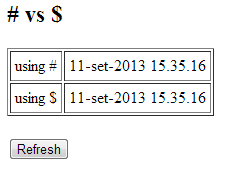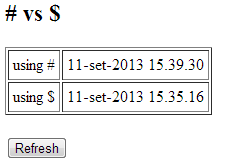# # vs \$ in the computed expressions in the xpages

In the xml code of the xpages the computed expressions begin with the character “#” or with the character “\$”, ie they are one of the following types:

• value=”#{[language]:[expression]}”
• value=”\${[language]:[expression]}”

where [language] is the name of the language, for example javascript and [expression] is the expression to compute.

The question is “what is the difference between the two cases?”.
To answer this question, consider the following example:

this xpage contains two Computed Field controls that display the current date and a button to refresh the page.
If you open the xpage with a browser it looks like the following figure:with equal times.
Then if you perform a refresh using the button, the page becomes:with different times because only the computed field with the character “#” is updated.
So now you can answer the question above:

• value=”#{[language]:[expression]}” is computed at each update of the page
• value=”\${[language]:[expression]}” is computed only once at the first loading of the page
• In the designer client you get the two cases selecting the property “Value” of the Computed Field control “Compute dynamically” o “Compute on page load” as in figure:

This site uses Akismet to reduce spam. Learn how your comment data is processed.